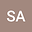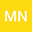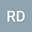A high-order predictor-corrector method for initial value problems with fractional derivative involving Mittag-Leffler kernel: epidemic model case study
•••• +1Mohd Salmi Md Noorani
Universiti Kebangsaan Malaysia Fakulti Sains dan Teknologi
Author ProfileRedouane Douaifia
Laboratory of Mathematics, Informatics and Systems (LAMIS), Larbi Tebessi University, Tebessa, Algeria
Author ProfileSalem Abdelmalek
University of Tebessa Faculty of Exact Sciences and Natural and Life Sciences
Author Profile## Abstract

In this paper, we propose a numerical scheme of the predictor-corrector type for solving nonlinear fractional initial value problems, the chosen fractional derivative is called the Atangana-Baleanu derivative defined in Caputo sense (ABC). This proposed method is based on Lagrangian quadratic polynomials to approximate the nonlinearity implied in the Volterra integral which is obtained by reducing the given fractional differential equation via the properties of the ABC-fractional derivative. Through this technique, we get corrector formula with high accuracy which is implicit as well as predictor formula which is explicit and has the same precision order as the corrective formula. On the other hand, the so-called memory term is computed only once for both prediction and correction phases, which indicates the low cost of the proposed method. Also, the error bound of the proposed numerical scheme is offered. Furthermore, numerical experiments are presented in order to assess the accuracy of the new method on two differential equations. Moreover, a case study is considered where the proposed predictor-corrector scheme is used to obtained approximate solutions of ABC-fractional generalized SI (susceptible-infectious) epidemic model for the purpose of analyzing dynamics of the suggested system as well as demonstrating the effectiveness of the new method to solve systems dealing with real-world problems.
06 May 2022Submitted to Mathematical Methods in the Applied Sciences
11 May 2022Assigned to Editor
11 May 2022Submission Checks Completed
13 May 2022Reviewer(s) Assigned